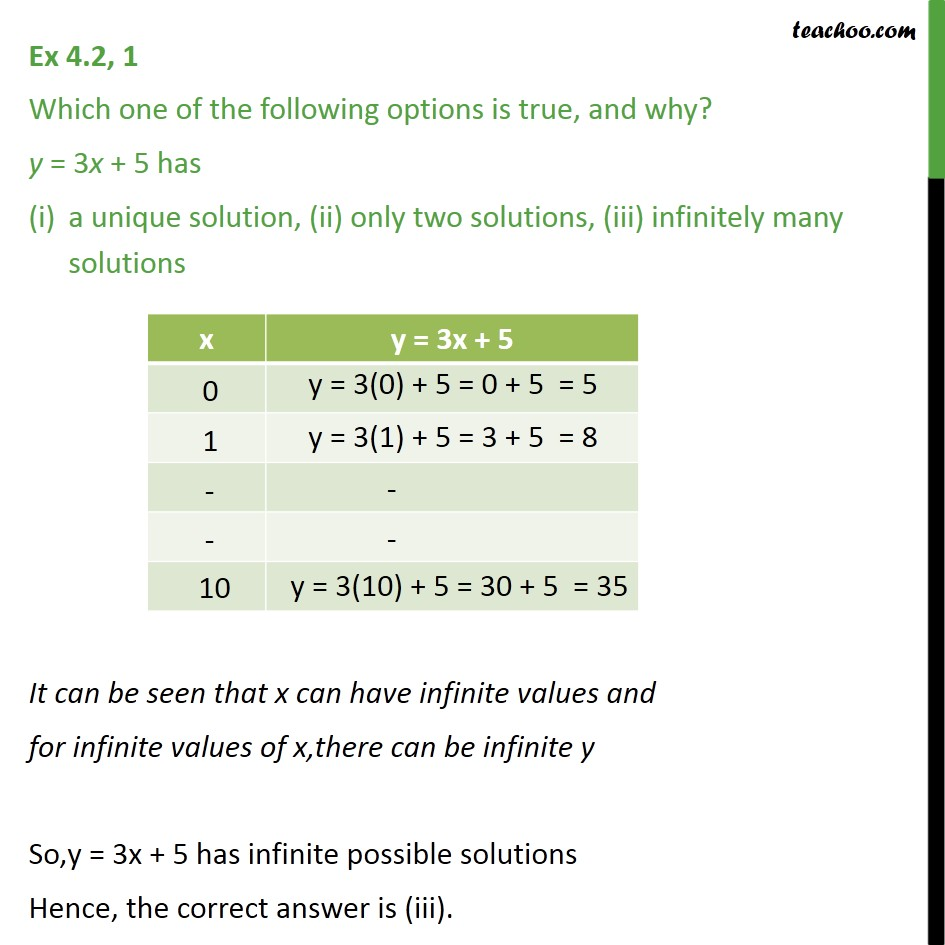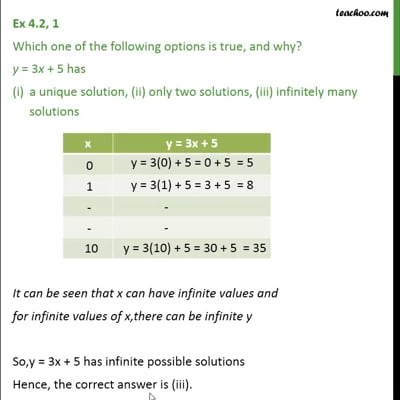Ex 4.2

Chapter 4 Class 9 Linear Equations in Two Variables
Serial order wiseThis video is only available for Teachoo black users

Maths Crash Course - Live lectures + all videos + Real time Doubt solving!

### Transcript

Ex 4.2, 1 Which one of the following options is true, and why? y = 3x + 5 has a unique solution, (ii) only two solutions, (iii) infinitely many solutions It can be seen that x can have infinite values and for infinite values of x,there can be infinite y So,y = 3x + 5 has infinite possible solutions Hence, the correct answer is (iii).